# Sampling at the Nyquist Rate

August 1, 2019

In Example 1, the time waveform x(t) was sampled at 𝖥s = 4 × 𝖿h. The resulting DTFT spectral images were spread out in the DTFT frequency domain.

By examining these results, it is apparent that the minimum sample rate is 𝖥s = 2 × 𝖿h. Anything less would result in the overlap of spectral images.

The 𝖥s = 2  × 𝖿h rate is called the Nyquist rate. Example 5 is similar to Example 4, but the sample rate 𝖥s is reduced to the Nyquist rate.

### Frequency Content of a Waveform Sampled at Nyquist Rate (Ex. 5)

Given the time waveform illustrated in Figure 2.1:

(1)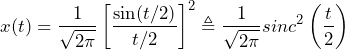The Fourier transform 𝖷̃(𝜔) is the triangle function illustrated in Figure 2.3 with the highest frequency component of 𝖿h = 1/2𝜋 Hz.

The waveform x(t) sampled at 𝖥s ≜ 2 × 𝖿h = 4 × 1/2𝜋 = 1/𝜋 gives a sample period of 𝜏 = 1/𝖥s = 𝜋 and is illustrated in Figure 2.5.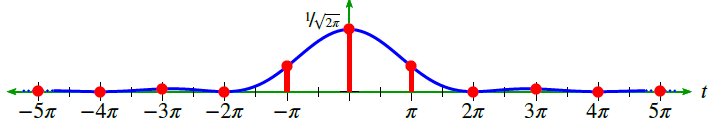Figure 2.5. Sample sequence.

Using this sample rate and Theorem 1, a DTFT 𝖸̃(𝜔) of y(n) is expressed as:

(2)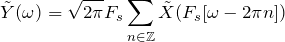(3)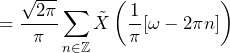### Conclusion

• 𝖷̃(𝜔) repeats every 2𝜋 (this is true of all DTFTs)
• 𝖷̃(𝜔) reaches 0 when (1/𝜋) 𝜔 = 1 or 𝜔 = 𝜋
• The height of each triangle is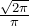or about 0.798 (see Figures 2.6 and 2.7)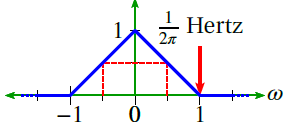Figure 2.6. Fourier transform.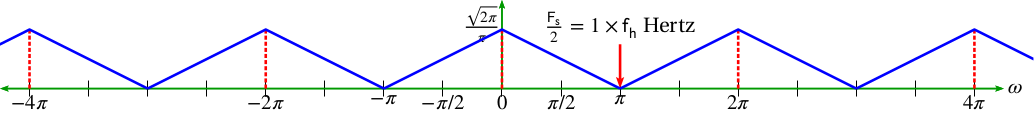Figure 2.7. DTFT.